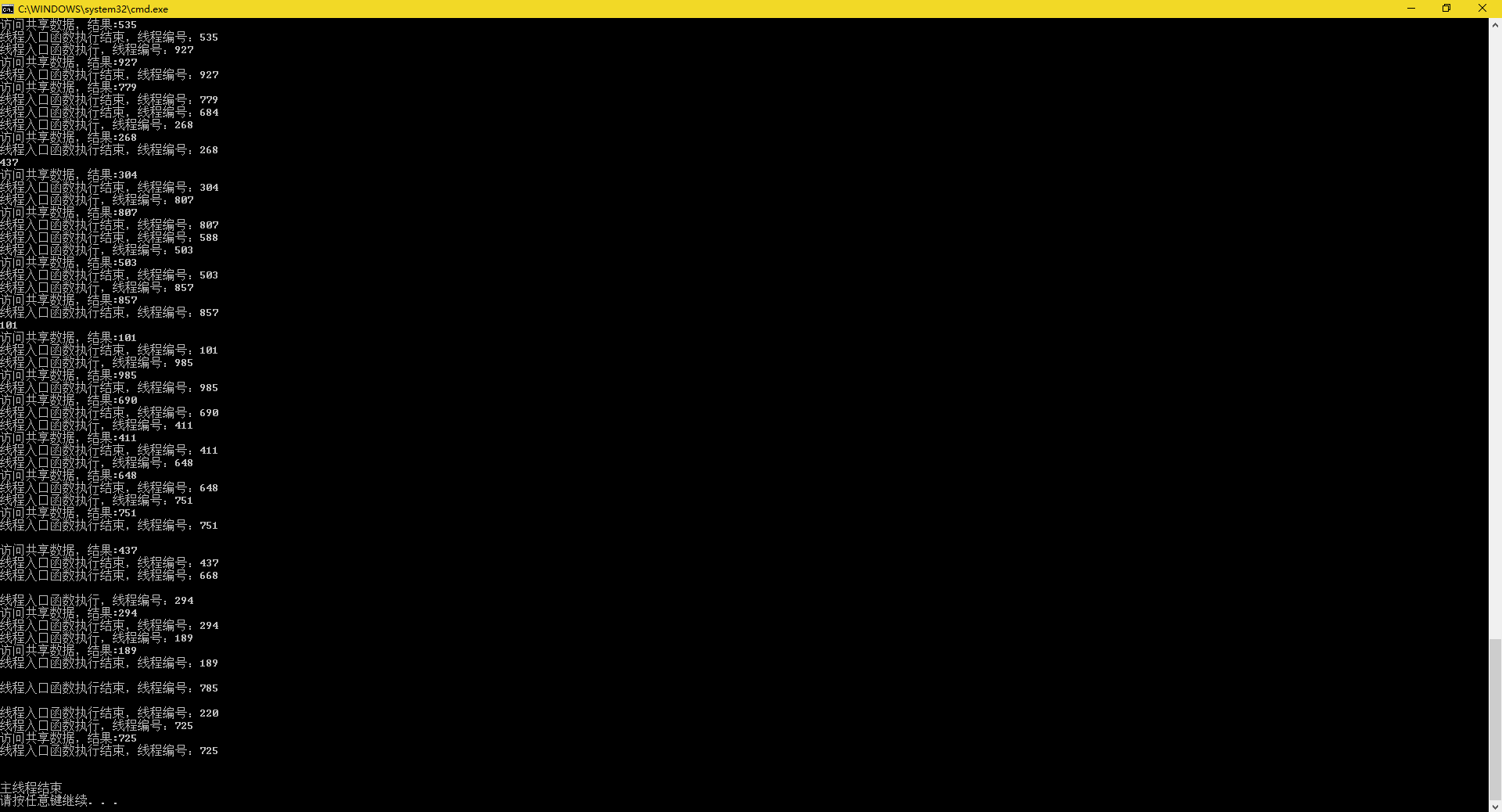# 数据共享

#include <vector>
#include <iostream>

using namespace std;
static int share;

{
cout << "线程入口函数执行，线程编号：" << threadCount << endl;
cout << "访问共享数据，结果:" << share[threadCount] << endl;
cout << "线程入口函数执行结束，线程编号：" << threadCount << endl;
}

int main()
{
for (int i = 0; i < 1000; i++)
{
share[i] = i;
}

for (int i = 0; i < 1000; i++)
{
}
{
iter->join();
}
cout << "主线程结束" << endl;
return 0;
}### 消息队列：

#include <list>
#include <iostream>

using namespace std;

//队列遵循先入先出原则
class MsgQueue
{
public:
//插入消息队列
void InMsgQueue()
{
for (int i = 0; i < 10000; i++)
{
msgQueue.push_back(i);
cout << "新的请求入队，请求码：" << i << endl;
}
}
//从队列输出
void OutMsgQueue()
{
for (int i = 0; i < 10000; i++)
{
if (!msgQueue.empty())
{
cout << "请求出队，请求码：" << msgQueue.front() << endl;
msgQueue.pop_front();
}
else
{
//消息队列为空
cout << "无请求" << endl;
}
}
}
private:
//使用链表，头尾增删效率更高
list<int> msgQueue;
//这里的int就是消息的类型
};

int main()
{
MsgQueue queue;
InQueueObj.join();
OutQueueObj.join();

cout << "主线程结束" << endl;
return 0;
}


# 互斥锁

### lock()和unlock()

mutex my_mutex;
my_mutex.lock();
//读写操作
my_mutex.unlock();


class MsgQueue
{
public:
//插入消息队列
void InMsgQueue()
{
for (int i = 0; i < 10000; i++)
{
my_mutex.lock();
msgQueue.push_back(i);
my_mutex.unlock();
cout << "新的请求入队，请求码：" << i << endl;
}
}
void OutMsgQueue()
{
for (int i = 0; i < 10000; i++)
{
if (!msgQueue.empty())
{
my_mutex.lock();
cout << "请求出队，请求码：" << msgQueue.front() << endl;
msgQueue.pop_front();
my_mutex.unlock();
}
else
{
//消息队列为空
cout << "无请求" << endl;
}
}
}
private:
list<int> msgQueue;//使用链表，头尾增删效率更高
mutex my_mutex; //互斥量对象
};


### lock_guard类

	void InMsgQueue()
{
for (int i = 0; i < 10000; i++)
{
lock_guard<mutex> my_guard(my_mutex);
msgQueue.push_back(i);
cout << "新的请求入队，请求码：" << i << endl;
}
}


# 死锁

mutex1，mutex2，

A和B

A需要先mutex1，后mutex2
B需要先mutex2，后mutex1

1. 线程A执行，它先锁mutex1成功，它现在去锁mutex2
2. 处理机调度，上下文切换
3. 线程B执行，它先锁mutex2 成功 ，它现在去锁mutex1
4. 处理机调度，上下文切换
5. 线程A无法得到mutex2中的东西，阻塞在mutex2.lock(）
6. 处理机调度，上下文切换
7. 线程B无法得到mutex1中的东西，阻塞在mutex1.lock(）

### 死锁的解决方案:

	void InMsgQueue()
{
for (int i = 0; i < 10000; i++)
{
my_mutex1.lock();
my_mutex2.lock();
msgQueue.push_back(i);
my_mutex2.unlock();
my_mutex1.lock();
cout << "新的请求入队，请求码：" << i << endl;
}
}
void OutMsgQueue()
{
for (int i = 0; i < 100000; i++)
{
//顺序不同，此处发生死锁
my_mutex2.lock();
my_mutex1.lock();
if (!msgQueue.empty())
{

cout << "请求出队，请求码：" << msgQueue.front() << endl;
msgQueue.pop_front();
my_mutex1.unlock();
my_mutex2.unlock();
}
else
{
//消息队列为空
cout << "无请求" << endl;
my_mutex1.unlock();
my_mutex2.unlock();
}
}
}


# std::lock()函数模板

std::lock(my_mutex1,my_mutex2);


using namespace std;
void InMsgQueue()
{
for (int i = 0; i < 10000; i++)
{
lock(my_mutex1,my_mutex2);
msgQueue.push_back(i);

cout << "新的请求入队，请求码：" << i << endl;
}
}


### unique_lock取代lock_guard

unique_lock是一个类模板，工作中一般使用lock_guard已经足够了，unique_lock比lock_guard要灵活很多，有更多的功能，但效率上要差一点，内存也要占多一点

### std::try_to_lock

try_to_lock是一个unique_lock的参数。

unique_lock<std::mutex> my_unique(my_muteax,std::try_to_lock);


void InMsgQueue()
{
for (int i = 0; i < 10000; i++)
{
unique_lock<mutex> my_unique(my_mutex, try_to_lock);
if (my_unique.owns_lock())
{
msgQueue.push_back(i);
cout << "新的请求入队，请求码：" << i << endl;
}
else
{
doSomethingelse();
}
}
}


### defer_lock

defer_lock是一个unique_lock的参数

## unique_lock的成员函数

unique_lock的成员函数有四个：
lock(),unlock(),try_lock(),release()

release():返回它所管理的mutex对象指针，释放对它的绑定，使之不再和mutex有关系

release的返回值证明了unique_lock本质上一个指向mutex的指针，这也暗示unique_lock和mutex彼此之间是一一对应的。

unique_lock的成员函数使我们能更灵活的运用锁，不用再让锁的范围依赖于对象的作用域，能够提前解锁，这里提一个概念，就是锁的粒度。

### unique_lock的所有权传递

std::unique_lock<std::mutex> my_unique1(my_mutex);
//else...
//...
std::unique_lock<std::mutex> my_unique2(std::move(my_unique1));


std::unique_lock<std::mutex> rtn_unique()
{
std::unique_lock<std::mutex> temp(my_mutex);
return temp;
}


04-17417511-281061
11-18330
03-22136
01-201万+
09-18934
03-17
12-022万+
05-305335
03-101万+
09-05154
06-11322
09-0993
03-0184
06-28202
03-22684
01-251065